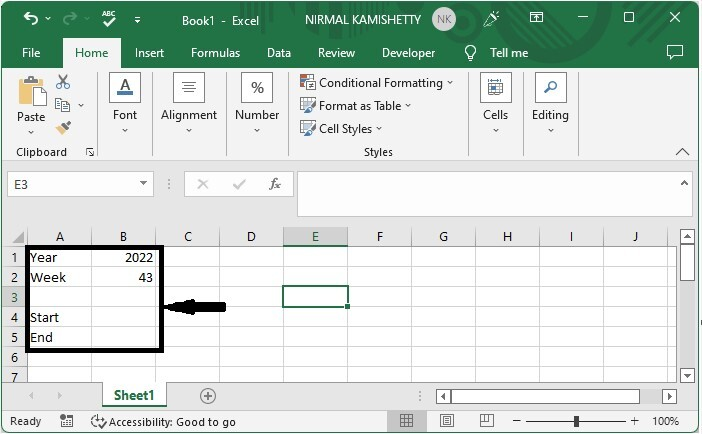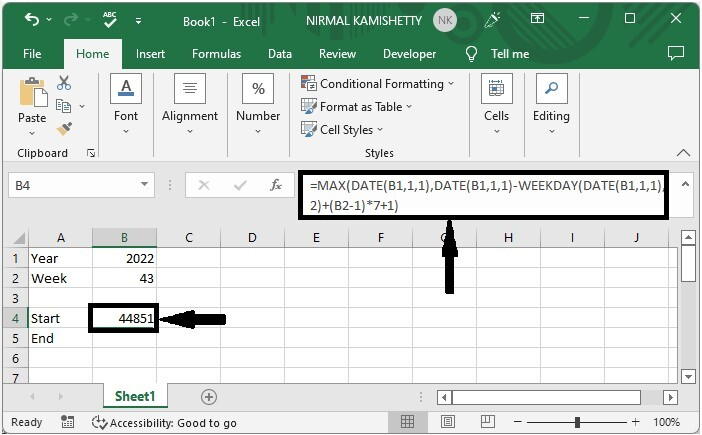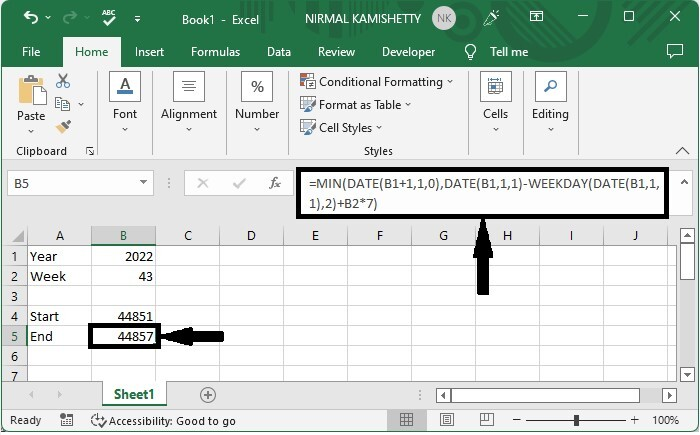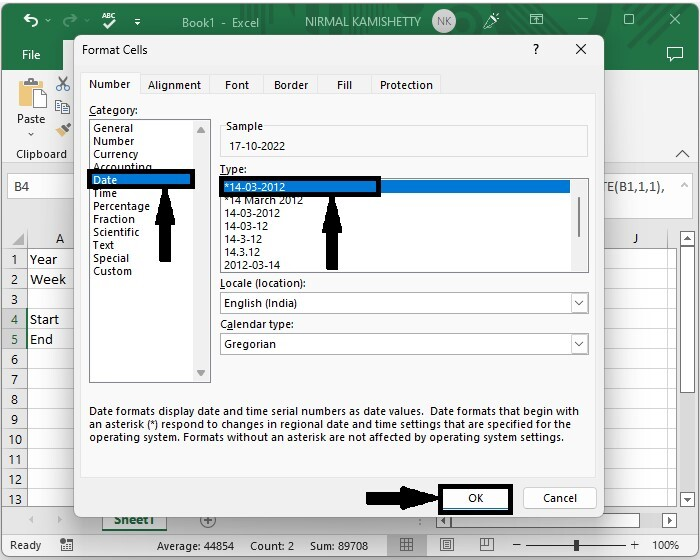# How to Convert Week Number to Date or Vice Versa in Excel?

Have you ever wondered if there is any feature in Excel from which we can find the start and end dates of a week in any year without using the calendar? There is no direct way, but we can solve this task in Excel using the formulas supported by the programme.

Read this tutorial to learn how you can convert a number to a date or a date to a week number in Excel.

## Converting a Week Number to Date in Excel

Here we will first use the formulas to get the start and final data. Let's look at a simple procedure for converting a week number to a date in Excel.

### Step 1

Let us consider an Excel sheet where the data in the sheet is similar to the data shown in the below image. We need to determine the start and end dates of the specified week in the given year.Now to get the start date In our case, cell B4, click an empty cell and enter the formula =MAX(DATE(B1,1,1),DATE(B1,1,1)-WEEKDAY(DATE(B1,1,1), 2)+(B2-1)*7+1) and press enter. In the formula, B1 is the address of our year, and B2 is the address of the week number.

Empty cell > Formula > Enter### Step 2

Now to get the end data, click on the cell B5 and enter the formula as

=MIN(DATE(B1+1,1,0),DATE(B1,1,1)-WEEKDAY(DATE(B1,1,1),2)+B2*7)

and click enter.

Empty cell > Formula > EnterAs we can clearly see, the results are in the form of integers, so we need to convert them into date format.

### Step 3

To do so, select the values, then right-click and select view code, click on date, then click OK to complete our process.

Select data > Right click > Format cells > Date > OKIf we need to find the week number of any date, we use the formula =WEEKNUM(B1,1), where B1 is the address of the date on the sheet.

## Conclusion

In this tutorial, we used a simple example to demonstrate how you can convert a week number to a date or vice versa in Excel.

Updated on: 07-Mar-2023

15K+ Views